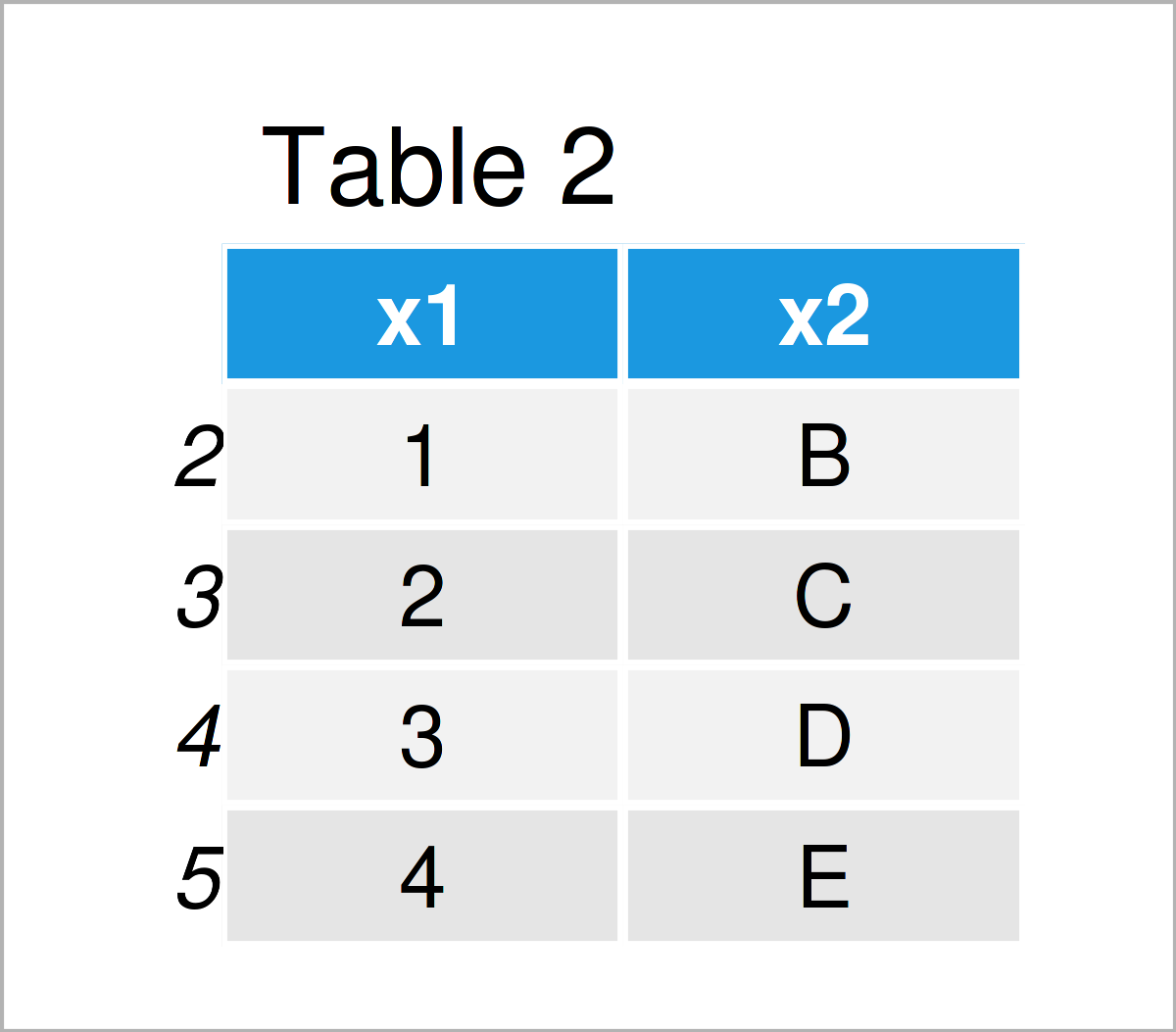# Remove Values Lesser & Greater than 5th & 95th Percentiles in R (2 Examples)

On this page, I’ll show how to drop values lesser and greater than the 5th and 95th percentiles in R programming.

The article will consist of this:

Important note: Removing certain values (i.e. outliers) in data sets is a very controversial topic. Make sure that the removal of any observations is theoretically justified. You can find more info on outlier detection and removal here.

So now the part you have been waiting for – the exemplifying R syntax…

## Example 1: Remove Values Below & Above 5th & 95th Percentiles

This example shows how to delete values above and below a certain percentile in a numeric vector object.

For this, we first have to create an example vector:

```x <- c(1, 3, 7, 100, 5, 5, - 987, 6)                   # Create example vector
x                                                      # Print example vector
#     1    3    7  100    5    5 -987    6```

Next, we have to calculate the 5th and 95th percentiles of this vector using the quantile function:

```x_quantiles <- quantile(x, c(0.05, 0.95))              # Calculate 5th & 95th percentiles
x_quantiles                                            # Print 5th & 95th percentiles
#      5%     95%
# -641.20   67.45```

In the next step, we can use those percentile thresholds to subset our vector object:

```x_subset <- x[x > x_quantiles &                     # Drop values below/above percentiles
x < x_quantiles]
x_subset                                               # Print subset of values
#  1 3 7 5 5 6```

The previous R code has created a new vector object called x_subset, where we have retained only values greater than the 5th percentile and lesser than the 95th percentile.

## Example 2: Remove Data Frame Rows Below & Above 5th & 95th Percentiles

In this example, I’ll show how to remove rows of a data frame where the value in a certain column is below or above the 5th & 95th percentile.

First, let’s create some example data:

```data <- data.frame(x1 = c(999, 1:4, - 777),            # Create example data frame
x2 = LETTERS[1:6])
data                                                   # Print example data frame```Table 1 shows the output of the previous R programming code – A data frame containing two columns.

Let’s assume that we want to remove the rows with the largest and smallest values in the column x1. Then, we first have to identify the 5th and 95th percentile of this variable:

```data_x1_quantiles <- quantile(data\$x1, c(0.05, 0.95))  # Calculate 5th & 95th percentiles
data_x1_quantiles                                      # Print 5th & 95th percentiles
#      5%     95%
# -582.50  750.25```

In the next step, we can remove all rows where the value in the column x1 is too small or too large:

```data_subset <- data[data\$x1 > data_x1_quantiles &   # Drop rows below/above percentiles
data\$x1 < data_x1_quantiles, ]
data_subset                                            # Print subset of values```After executing the previously shown R programming code the data frame subset without outliers shown in Table 2 has been created.

## Video & Further Resources

I have recently released a video on my YouTube channel, which shows the R syntax of this article. You can find the video below:

Please accept YouTube cookies to play this video. By accepting you will be accessing content from YouTube, a service provided by an external third party.If you accept this notice, your choice will be saved and the page will refresh.

Furthermore, you may want to read some of the other articles on https://www.statisticsglobe.com/. A selection of tutorials about topics such as graphics in R, missing data, and vectors can be found below:

At this point you should know how to remove values lesser and greater than the 5th and 95th percentiles in R. If you have any additional questions, kindly let me know in the comments.

Subscribe to the Statistics Globe Newsletter